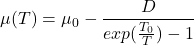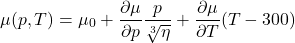# Modulus of Rigidity – Equation, Steel Selection, and Values

#### Engineering

The modulus of rigidity is the ratio of shear stress to shear strain. Thus, it measures the ability of the material to withstand distortion. However, the rigidity of the material varies at different temperatures and pressures. The main value of the modulus of rigidity is a description of the material hardness and insight into the point where material deformation occurs. In this article, you will learn the modulus of rigidity equation, its applicability to steel, and common values.

## Modulus of Rigidity Equation

The Modulus of rigidity(G), which is sometimes referred to as the shear modulus, can be calculated simply by shear stress(τxy) over shear strain(γxy).Shear stress may be expanded into force(F) over the area(A) where the force acts. Shear strain may also expand into the transverse displacement(Δx) over the initial length of the area(l).There are also models that exist to predict this number for different metals, alloys, and plastics. These take into account temperature, pressure, and other factors to determine how the modulus of rigidity changes with the variation of these factors.

### The MTS Model

The MTS model is used mainly for plastics. This specific model only uses temperature and not pressure. The model takes in a few different variables to work, such as the shear modulus(μ0) when the temperature(T) is at 0K, and the flexural rigidity(D).### The SCG Model

Another model is the SCG(Steinberg-Cochran-Guinan) model, a pressure dependent model. This model accounts account for density(ρ), pressure(p) and temperature(T). The modulus of rigidity(μ0) is considered at (T = 300K, p = 0, η = 1).Where:### The NP Model

The last commonly used model is NP(Nadal-Le Poac) which is a variation of the SCG model. NP swaps the temperature dependence of the SGC for a part based on the Lindemann melting theory. Variables are temperature(T), pressure(p), the Lindemann constant(f), the atomic mass(m), and a material parameter(ζ). The modulus of rigidity(μ0) is considered at (T = 0K, p = ambient pressure).Where: